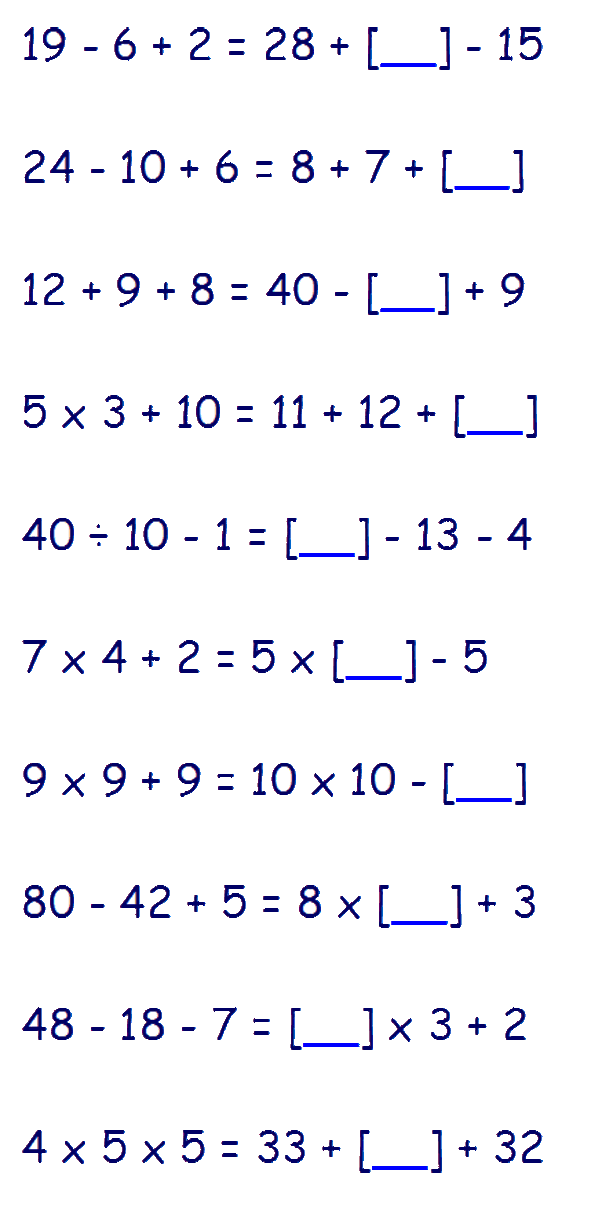# Type I - Complete the Calculation

With this type of question you are given a number equation (number sentence) with a number missing. Both sides of the equation need to have the same value.

You are required to find the missing number that will balance the equation.

If your child has already covered Algebra in maths, they should have no problem with this. It is also important that they understand Order of Operations (BODMAS or BIDMAS).

Lets look at an example:

## 4 x 3 + 3 = 6 + 4 + [_]

One side of the equation will be complete (normally the left) - use this to calculate the total value for the equation. In this case the left side is complete - 4 x 3 +3 = 15. So each side of the equation must equal 15. (Remember to do the calculations in the correct order - Divide - Multiply - Add - Subtract).

We now know that the right side of the equation must also total 15. Therefore the missing number can be found by 15 - 6 + 4 = 5

## 4 x 3 + 3 = 6 + 4 + 

The missing number is 5. Check this by putting the number into the equation and ensuring that both side of the equation give the same result. 4 x 3 + 3 = 15 and 6 + 4 + 5 = 15

### Verbal Reasoning Type I - 'Complete the Calculation' practice